# Areas of Combinations of Plane Figures

In our daily life activities, we have come across different types of figures in the form of various interesting designs. The designs may be a simple plane figure or sometimes it may be a combination of different plane figures. We know that the plane figures are two-dimensional figures which have area and perimeter. The different plane figures which we are already familiar with are square, circle, rectangle, triangle, and so on. In this article, we are going to learn how to find the area of combinations of plane figures with many solved examples.

Before discussing how to find the area of combinations of planes, first, we will have a quick recall of the area of different two-dimensional shapes.

1. Area of square = a2 Square units, where a is the side length of square
2. Area of rectangle = l × b Square units, where l is the length, b is the breadth
3. Area of circle = π × r2 Square units, where r is the radius of a circle.
4. Area of Triangle = ½ × b × h Square units, where b is the base and h is the height
5. Area of parallelogram = b × h Square units, where b is the base, h is the vertical height of the parallelogram.
6. Area of Trapezium = ½(a+b) × h Square units, where a and b are the length of the parallel sides and h is the height.

## How to Find the Areas of Combination of Plane Figures?

Go through the following examples to understand the method of finding the areas of combinations of plane figures.

Example 1:

Find the area of the shaded region in the given figure, where ABCD is a square whose side length is 14 cm.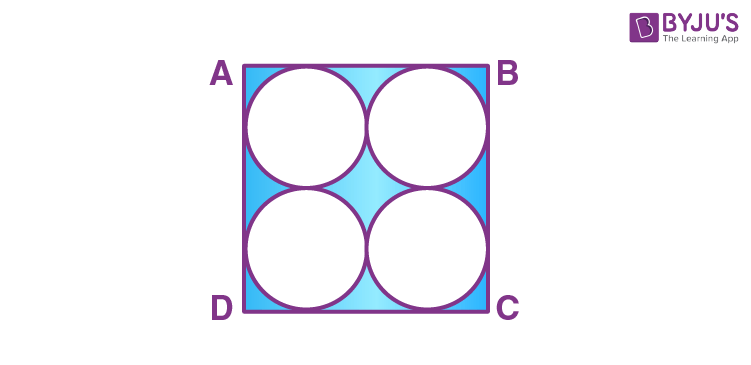Solution:

Given that ABCD is a square.

The side length of the square, a = 14 cm.

We know that the formula to find the area of a square is a2 square units.

Now, substitute a = 14 in the area of square formula, we get

Area of a square = 142 cm2

A = 14×14 cm2

A = 196 cm2

Therefore, the area of a square is 196 cm2.

The diameter of each circle = 14/2 = 7 cm

Therefore, the radius of each circle = 7/2 cm or 3.5 cm

The area of circle = πr2 square units.

Substitute π= 22/7 and r = 7/2 in the above formula, we get

Area of one circle = (22/7)×(7/2)×(7/2) square units

Area of one circle = 77/2 cm2.

In the given figure, there are 4 circles.

Thus, the area of 4 circles = 4(77/2) = 154cm2.

Therefore, the area of shaded region = Area of the square – Area of 4 circles.

Area of shaded region = (196 – 154) cm2 = 42 cm2.

Therefore, the area of the shaded region is 42 cm2.

Example 2:

Compute the area of the shaded region as shown in the figure, where ABCD is a square whose side length is 10 cm and semicircles are drawn with each side of a square as diameter. (Use: The value of π is 3.14.)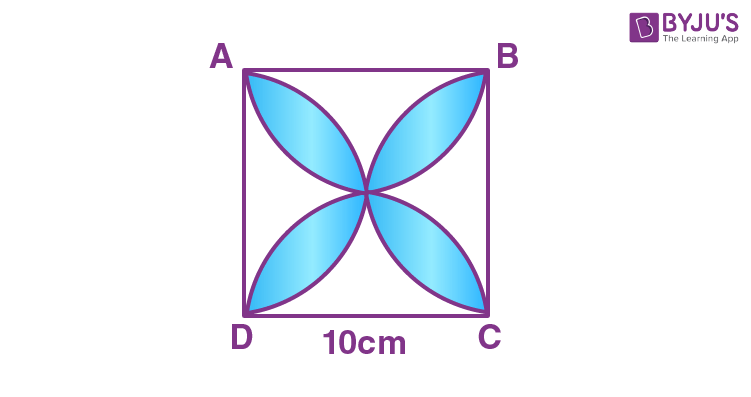Solution:

From the given figure, first mark the unshaded region as I, II, III, and IV as shown below: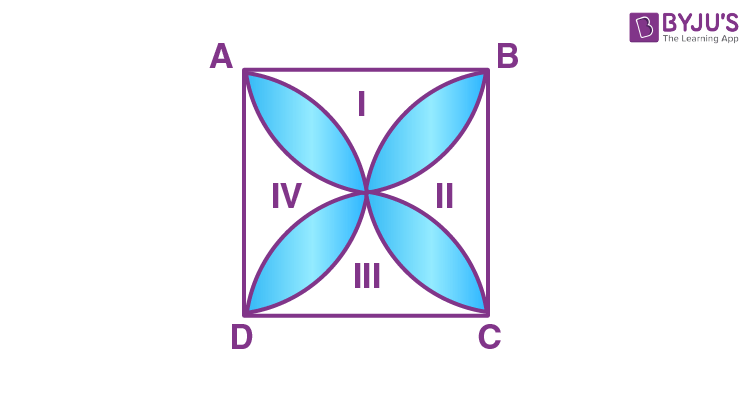First, find the sum of areas I and III and similarly, find the sum of areas II and IV.

Sum of Area I and Area III:

Area of I + Area of III = Area of ABCD – Areas of two semicircles with each of radius 5 cm.

= (10)2 – (2× (½)× π×52) cm2

= 100 – 3.14(25) cm2

= 100 – 78.5 cm2

= 21.5 cm2.

Therefore, the sum of the area of I and area III is 21.5 cm2.

Sum of Area II and Area IV:

Area of II + Area of IV = Area of ABCD – Areas of two semicircles with each of radius 5 cm

Area of II + Area of IV = 21.5 cm2.

Therefore, the area of shaded region = Area of square – Area of (I + II + III + IV) square units.

Area of Shaded region = 100 – (21.5+ 21.5) cm2

= 100 – 43 cm2

= 57 cm2

Therefore, the area of the shaded region = 57 cm2.

### Practice Problems

Solve the following problems:

1. Find the area of the shaded region for the following figure, where ABCD is a square whose side length is 14 cm and APD and BPC are the semicircles.
2.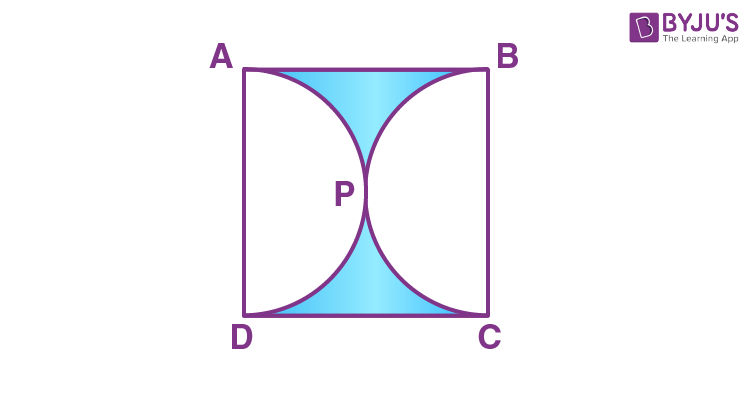3. Find the area of the shaded for the figure given below, where ABCD is a square of 14cm. With centres A, B, C and D, four circles are drawn such that each circle touches two of the remaining three circles.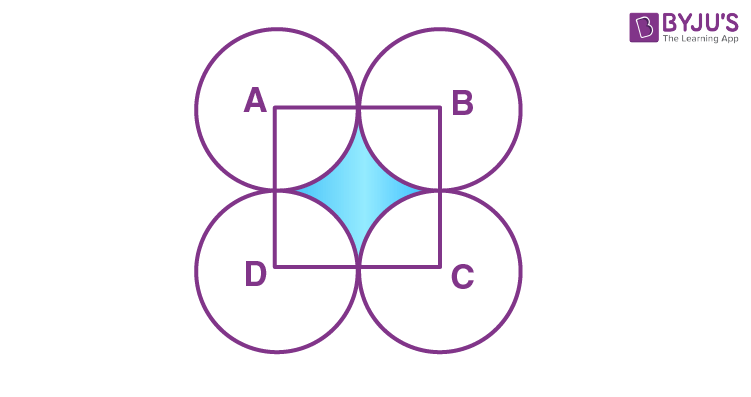## Frequently Asked Questions on Area of Plane Figures

### What are plane figures?

The plane figures are two-dimensional figures, such that the collection of all the points should be on the plane surface.

### Give a few examples of plane figures.

A few examples of plane figures are square, rectangle, circle and triangle.

### Mention the difference between a plane figure and a solid figure.

The plane figure is a two-dimensional figure and it has only two dimensions. Whereas, the solid figure is a three-dimensional figure and has three dimensions.

### Is a circle a plane figure?

Yes, a circle is a plane figure, as it is a two-dimensional figure.

### Is a cuboid a plane figure?

No, a cuboid is not a plane figure, since it is a three-dimensional figure.